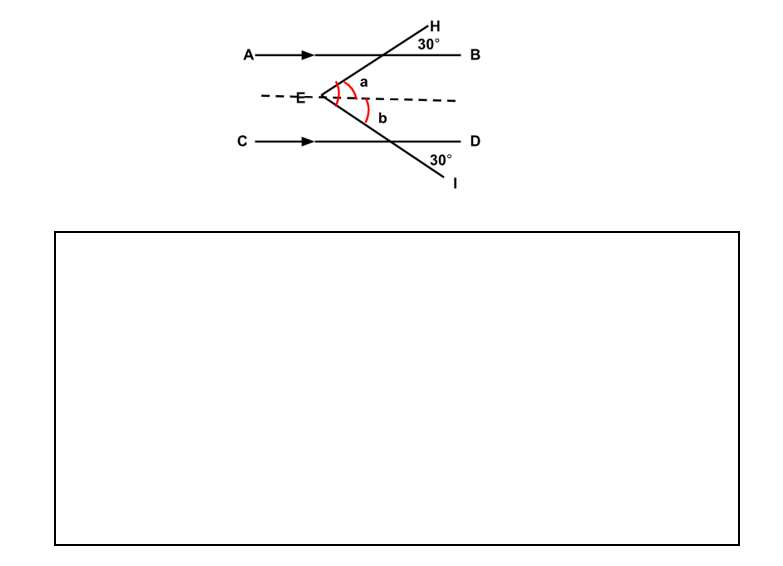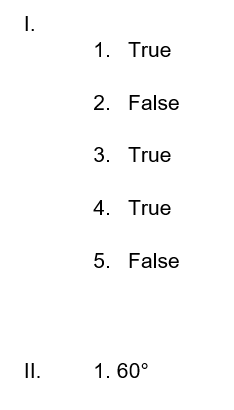In geometry, parallel lines are coplanar, straight lines that don’t cross at any point. In the same three-dimensional space, parallel planes are any planes that never intersect. Curves that are parallel to one another and maintain a set minimum distance from one another are known as parallel curves.

What is the “Parallel Lines Worksheet Grade 8”?

This worksheet provides detailed information about Parallel Lines.

## What is a Parallel Line?

Parallel lines are any two or more lines that all lie in the same plane and never cross one another. They are equally spaced apart and have the same incline. In this article, let’s learn more about parallel lines.

Instructions on how to use the “Parallel Lines Worksheet Grade 8.”

Study the concept and examples given and try to solve the given exercises below.

## Conclusion

There are two prerequisites for parallel lines: first, they must be spaced apart from one another, and second, they must not cross at any point. Only you have the authority to declare lines parallel if both conditions are met.

If you have any inquiries or feedback, please let us know.

Parallel Lines, no matter how far we extend them, parallel lines are straight lines that never intersect.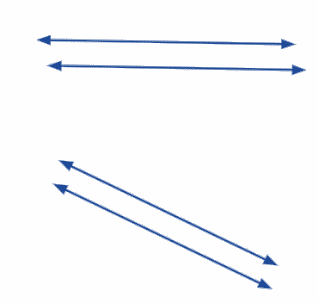Parallel Lines Properties

With the help of the essential characteristics listed below, parallel lines can be easily identified.

• Straight lines parallel to one another are those whose distances from one another are constant.
• No matter how far apart they are from one another in either direction, parallel lines will never cross.

Parallel Lines Equation

In most cases, a straight line’s equation is expressed in the slope-intercept form, represented by the equation y = mx + b, where m denotes the slope and b is the y-intercept. The slope or gradient of the line and its steepness are determined by the value of the variable “m.”

For example:  Find the value of x.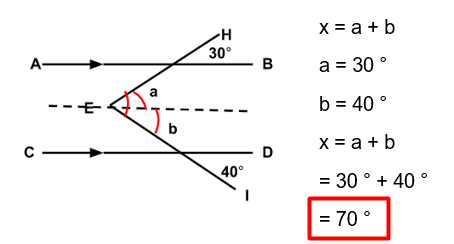## Worksheet

1. Identify whether it is true or false.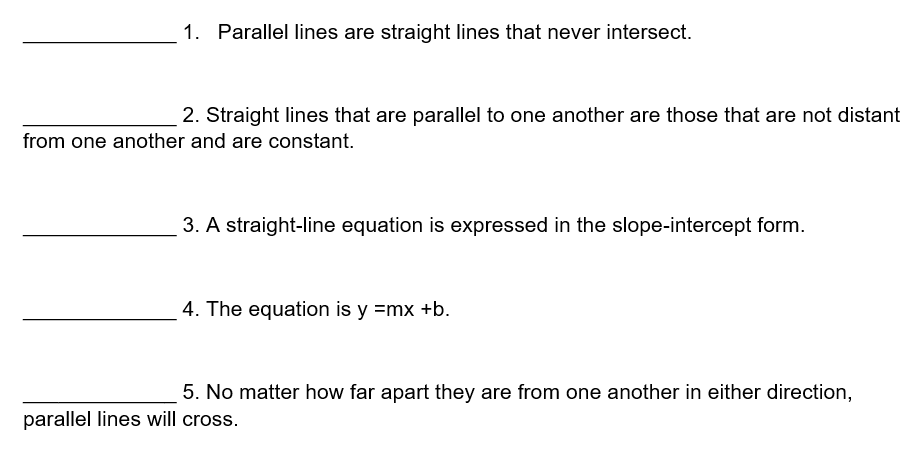1. Find the value of x.